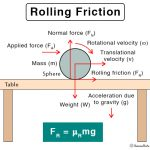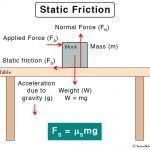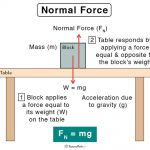Home / Physics / Electrostatic Force

# Electrostatic Force

## What is the Electrostatic Force?

The electrostatic force is the force of attraction or repulsion between two charged particles. It is also called Coulomb’s force or Coulomb’s interaction. For example, the force between the protons and electrons in an atom is electrostatic and is responsible for the atom’s stability. In chemistry, the electrostatic bonding force is important and binds an ionic molecule.

The laws of electrostatics were discovered by French physicist Charles Augustin de Coulomb in 1785 and are known as Coulomb’s law.

## Laws Governing Electrostatic Force

### Coulomb’s Law

The electrostatic force between two charged particles can be quantified by Coulomb’s law. It is usually applied to point charges and gives a relationship among the electrostatic force, the magnitude of the charges, and separation distance. According to this law, the force between the two particles is,

• Directly proportional to the product of the magnitude of the charges
• Inversely proportional to the square of the distance between the two charges

Suppose the two charged particles are brought close to one another. There will be an attraction if the charges are opposite, i.e., if one is positive and the other negative. On the contrary, the charges will repel if both of them are positive or negative. To summarize, like charges repel and unlike charges attract.

### Electrostatic Force Equation

Let us assume that q1 and q2 are the amounts of charges on the two particles separated by a distance r. According to Coulomb’s law, the electrostatic force between the two charges is given by the following equation:

F = k q1 q2/r2

Electrostatic force unit: N (Newton).

Here, k is called Coulomb’s constant. Its value is 9 x 109 N.m2.C-2. Generally, q1 and q2 can be positive or negative. When two opposite point charges are placed close to each other, the direction of force is positive to negative. The magnitude is merely the value of F without the sign. According to the above equation, F vanishes when r → ∞. Hence, at an infinitely large distance, the electrostatic force is zero. Technically, the range of F is infinite.

The work done W by the force F on a particle is the product of the force and the displacement d.

W= F x d

The work done in displacing the particle from one position to another is independent of the path taken. Hence, the electrostatic force is conservative.

## Properties of Electrostatic Force

Here are some facts and characteristics of the electrostatic force.

• Like charges repel, and opposite charges attract
• Directly proportional to the product of two point-charges
• Inversely proportional to the distance of separation between the charges
• Acts along the line joining the two charges

## Examples of Electrostatic Force in Daily Life

The electrostatic force is felt in everyday life situations. Here are a few examples, along with images.

• Rubbing of clouds generate charges. These charges will neutralize by passing through the atmosphere until they reach the neutral ground. We perceive this as lightning.
• After combing, if we bring the comb close to a piece of paper, there is a force of attraction between them.
• A silk shirt clings on to the body because of charged particles on the shirt. The same applies to a woolen sweater when taking off.
• Getting out of a car on a warm, dry day and touching the door give us charges.
• Grains of sugar are attracted to the inside surface of a container due to electrostatic forces.

## Applications of Electrostatic Force

Here are a few common applications of the electrostatic force.

• Photocopier
• Laser and ink-jet printers
• Van der Graaff generator
• Smoke precipitator and
• CCD camera

## Difference between Electrostatic and Gravitational Forces

The electrostatic force and gravitational force are both fundamental forces of nature. There are a few similarities between the two as both follow inverse-square law. However, there are also some critical differences.

## Electrostatic Force Problems and Solutions

P.1. What is the ratio of electrostatic force and gravitational force of two electrons?

Soln. The ratio of electrostatic force to gravitational force of two electrons is given by

FE/FG = ke2/Gme2

= 9 x 109 N.m2.C-2x (1.6 x 10-19)2 C2/6.67 x 10-11 N.m2.kg-2x (9.1 x 10-31)2 kg2

= 4 x 1042

Therefore, the relationship between the gravitational force and the electrostatic force is

FE = 4 x 1042 FG

P.2. If the electrostatic force between two charges is 124 N. What is the distance of separation between the charges, if the charges are 4 μC and 9 μC? Given k = 9 x 109 N m2.C-2.

Soln. We shall use the following equation.

F = k q1 q2/r2

Given, q1 = 4 x 10-6 C, q2 = 9 x 10-6 C, F = 124 N and k = 9 x 109 N m2.C-2. Putting these values,

124 N =9 x 109 N m2.C-2 x 9 x 10-6 C x 4 x 10-6 C / r2

Or, r2 = 9 x 109 N m2.C-2 x 9 x 10-6 C x 4 x 10-6 C / 124 N

= 0.00261 m2

Or, r = 0.05 m

Article was last reviewed on Monday, November 16, 2020

### Related articlesRolling FrictionSliding FrictionStatic FrictionNormal Force